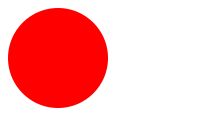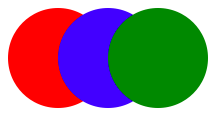# Award-Winning FjordsThomas Reynolds

## Canvas Hit Tracking

NOTE: Code samples are in CoffeeScript

One of the most fundamental interactions of any game is the ability to click (or touch) an on-screen component to manipulate it. Sounds simple enough, but when building HTML5 Canvas-based games, you'd be wrong.

The HTML5 Canvas allows us to easily draw shapes and images, but it does not provide an object model. What this means is, when I write code to draw a circle:

``````ctx.arc(10, 10, 10, 0, Math.PI * 2) # Arcs are in Radians
ctx.fill()
``````That code will draw the circle on the canvas immediately, but there is no link between what is displayed and what code caused it to be drawn.

Let's encapsulate drawing a circle into an Object we can reuse. We will also create an array of Objects being displayed.

## A Simple Drawing Object

``````class Circle
constructor: (@opt={}) ->
draw: (ctx) ->
ctx.arc(
@opt.x      ? 10, # Default to 10
@opt.y      ? 10, # Default to 10
@opt.radius ? 10, # Default to 10
0, Math.PI * 2
)
ctx.fill()
``````

Which can be used like so:

``````c = new Circle(x: 50, y: 50, radius: 50)
``````

## The World: A Collection of Objects

To keep track of our objects for drawing, we'll use a simple array:

``````theWorld = {
items: []
draw: ->
canvasElement = document.getElementById("world")
ctx = canvasElement.getContext("2d")

# Clear previously drawn
ctx.clearRect(
0, 0,
canvasElement.width+1, canvasElement.height+1
)

# Draw everything
obj.draw(ctx) for obj in @items
}
``````

Now let's add our circle to the world:

``````theWorld.items.push(c)
``````

Finally, let's draw the world:

``````theWorld.draw()
``````

## Naive Hit Tracking

So, now that we have an object representing a circle that we can manipulate and redraw, how do we figure out if clicking (or touching) a specific point on the canvas will "hit" a specific object?

A first solution might involve Bounding Boxes. A Bounding Box is a rectangular container which our drawn object would fill. In the circle example above, the bounding box of a circle drawn at `x=50`, `y=50` and with a `radius=5` would be:

• Top left: { x: 0, y: 0 }
• Top right: { x: 100, y: 0 }
• Bottom left: { x: 0, y: 100 }
• Bottom right: { x: 100, y: 100 }

Let's add code to our Circle object to calculate this:

``````# class Circle
getBounds: ->
{
}
``````

Remember, HTML5 Canvas draws circles from their center. The above can be used like this:

``````c = new Circle(x: 50, y: 50, radius: 50)
c.getBounds() # => { top: 0, left: 0, width: 100, height: 100 }
``````

Now, using simple math, we can calculate whether a click is inside that bounding box. Let's add more to Circle:

``````# class Circle
didHit: (targetX, targetY) ->
b = @getBounds()
(
(b.top  <= targetY <= b.top  + b.height) &&
(b.left <= targetX <= b.left + b.width)
)
``````

Running a few hit tests should return expected results:

``````c.didHit(5,   5)   # => true
c.didHit(0,   0)   # => true
c.didHit(10,  10)  # => true
c.didHit(100, 100) # => false
``````

### The Problem With Bounding Boxes

Our choice of shapes, a circle, should give you a hint as to the issues with using bounding boxes for hit testing. Circles are not rectangles, as such, they will give "false" positives at the points inside their bounds, but outside their arcs such as: `x=0,y=0`. The problem is fourth magnified as soon as we start drawing complex shapes or using images with transparent regions.

### The Great Thing About Bounding Boxes

Fear not! The above code is not useless. HTML5 Canvas has the ability to clear specific portions of an already drawn canvas. For performance reasons, you'll want to clear and redraw only the portions that change rather than clearing the whole canvas (as our `theWorld` example does). The method for clear is `clearRect` and as you may guess, it takes a bounding box rectangle.

If we had drawn our circle, we could they clear its bounding box like this:

``````# class Circle
clear: (ctx) ->
b = @getBounds()
ctx.clearRect(
b.left,
b.top,
b.left + b.width,
b.top  + b.height
)
``````

That said, let's move on to a pixel-perfect hit test. We'll need to refactor our Circle and world first.

## Offscreen Canvases

Another common HTML5 Canvas performance technique you'll encounter is using "offscreen canvases" to reduce the amount of drawing that drawing the whole world would require. Offscreen canvases are simply canvas elements which have not been added to the DOM. In this approach, each Object actually has its own canvas which contains only itself. Drawing the entire world simply copies each Object's canvas onto the main "onscreen canvas."

Let's look at a beefed-up Circle class which uses an offscreen canvas:

``````# class Circle
constructor: (@opt={}) ->
@canvas = document.createElement("canvas")
# @canvas.width  needs to be same side as world canvas
# @canvas.height needs to be same side as world canvas
@ctx = @canvas.getContext("2d")
@drawOffscreen()

drawOffscreen: ->
@ctx.arc(
@opt.x ? 10,
@opt.y ? 10,
0, Math.PI * 2
)
@ctx.fill()

# Note: Drawing the entire offscreen canvas onto the
# entire world canvas will get progressively slower as
# the dimensions of the canvas grows. Consider only
# drawing the image slice which represents this object's
# bounding box.
copyOffscreen: (ctx) ->
ctx.drawImage(@canvas, 0, 0)
``````

This time, we create an offscreen canvas for each Circle. `drawOffscreen` will render to that canvas and `copyOffscreen` will copy that image to the world canvas (passed in as ctx). `drawOffscreen` only needs to be called when the parameters which describe the Object change.

The world can now be drawn like so:

``````theWorld = {
items: []
draw: ->
canvasElement = document.getElementById("world")
ctx = canvasElement.getContext("2d")

# Clear Objects
obj.clear(ctx) for obj in @items

# Draw everything
obj.copyOffscreen(ctx) for obj in @items
}
``````

Finally, we're getting close to a modern, reusable and extensible system for managing Objects, how they clear themselves and how they draw themselves (offscreen and only on parameter change).

## Per-Object Canvas Hit Testing

Enough of the preamble, here's the meat. Now that we have an offscreen canvas for each Object that only contains itself, we can use that to check if we are "hitting" the drawn item. If there is a visible pixel at the point of the hit, then we return true.

``````# class Circle
didHit: (targetX, targetY) ->
imageData = @ctx.getImageData(targetX, targetY, 1, 1)
(imageData.data > 0)
``````

The `getImageData` method returns the RGBA values for the requested range of pixels on a canvas. In the above code, we ask for the target X/Y only. The resulting data is an array `[R, G, B, A]`. `imageData.data` is the alpha value of the pixel, if it is greater than zero then that means we drew something there and the hit test is true.

## Putting It All Together

Let's make an addition to `theWorld` to allow it to test all of its Objects and return only the items which we click on.

``````# theWorld
hitTestObjects: (targetX, targetY) ->
i for i in @items when i.hitTest(targetX, targetY)
``````

There we go, here's a complicated example:

``````c1 = new Circle(x: 50,  y: 50, radius: 50)
c2 = new Circle(x: 100, y: 50, radius: 50)
c3 = new Circle(x: 150, y: 50, radius: 50)

theWorld.push(c1)
theWorld.push(c2)
theWorld.push(c3)

theWorld.hitTestObjects(0,    0) # => []
theWorld.hitTestObjects(50,  50) # => [c1, c2]
theWorld.hitTestObjects(100, 50) # => [c1, c2, c3]
theWorld.hitTestObjects(150, 50) # => [c2, c3]
``````I could go further and try things out with arcs, empty stroked rectangles, donuts and semi-transparent images, but it would work exactly the same.

There's plenty of room for caching, memoization and other optimizations in this system, but conceptually this is common approach. I hope this was enlightening.